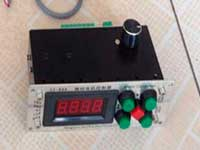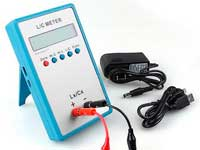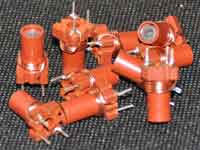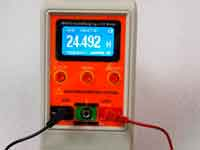Multilayer coil inductance calculator - 4.3 out of 5 based on 32 votes
Rating 4.31 (32 Votes)

# Online calculators

## Multilayer coil inductance calculator:

The most of the similar calculators work using Wheeler's formula, which has acceptable accuracy only when calculating the "optimal coil". Also, these calculators allow calculating just a ready-made coil. This calculator allows calculating the number of windings of the multilayer coil at the design stage. Inductance is calculated by the accurate algorithm using Maxwell's equations with elliptic integrals, see more... You can select measurement units - mm or inches, and also AWG or SWG wire number - the wire diameter will be determined automatically. You can download the cross-platform app Coil64 to calculate multilayer coil offline.

##### Calculate number of turnsENTER THE INPUT DATA:
 Select units: mm/cmmil/inch AWG → 000000000012345678910111213141516171819202122232425262728293031323334353637383940 SWG → 7/06/05/04/03/02/001234567891011121314151617181920212223242526272829303132333435363738394041424344454647484950

 L = mHμHnH – Required inductance D – Diameter of coil-former l – Length of winding d – Diameter of wire without insulation k – Diameter of wire with insulation Automatic

RESULT:
 N = – Number of turns n = – Number of Layers c – Winding thickness Lw – Required length of wire Ω =  Ohm – DC Resistance of coil

Another calculators:

Сomments from anonymous guests are enabled with moderation.

#### Coil Winding Machine###### US \$187.53
The system consists of individual components including a controller, a stepping motor, a foot switch, power adapter and output shaft. This design makes it very easy for you to integrate the system into your own project.

#### LC Meter LC200A###### US \$37.99
Measuring Capacitance Range:
0.01 pF - 100mF
Measuring Inductance Range:
0.001 uH - 100H
Measurement accuracy:1%.

#### RF Inductors###### US \$6.43
Variable Ferrite Core Inductor coil set 3.5Turns 25-100MHZ

#### Auto Ranging LCR Meter###### US \$63.07
Meter can measure 0.00pF to 100.00mF capacitance, 0.000uH to 100.00H inductance and 0.00? to 10.000M? resistance. Very suitable for small value capacitor and inductor measurement.